Sponsored Link •Python Buzz Forum
Slicing a list evenly with Python

0 replies on 1 page.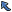Back to Topic List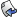Reply to this Topic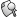Search Forum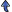Threaded View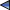Previous Topic Next Topic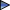Flat View: This topic has 0 replies on 1 page
Thomas Guest

Posts: 236
Nickname: tag
Registered: Nov, 2004

 Thomas Guest likes dynamic languages.
Slicing a list evenly with Python Posted: May 14, 2017 8:15 AMReply

 This post originated from an RSS feed registered with Python Buzz by Thomas Guest. Original Post: Slicing a list evenly with Python Feed Title: Word Aligned: Category Python Feed URL: http://feeds.feedburner.com/WordAlignedCategoryPython Feed Description: Dynamic languages in general. Python in particular. The adventures of a space sensitive programmer. Latest Python Buzz Posts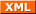Latest Python Buzz Posts by Thomas Guest Latest Posts From Word Aligned: Category Python

 AdvertisementHere’s a problem I came up against recently.

The task was to chop a list into exactly `n` evenly slized chunks. To give a little more context, let’s suppose we want to divide a list of jobs equally between `n` workers, where `n` might be the number of CPU cores available.

We can build the result by repeatedly slicing the input:

```def chunk(xs, n):
'''Split the list, xs, into n chunks'''
L = len(xs)
assert 0 < n <= L
s = L//n
return [xs[p:p+s] for p in range(0, L, s)]

```

This looks promising

```>>> chunk('abcdefghi', 3)
['abc', 'def', 'ghi']

```

but if the size of the list is not an exact multiple of `n`, the result won’t contain exactly `n` chunks.

```>>> chunk('abcde', 3)
['a', 'b', 'c', 'd', 'e']
>>> chunk('abcdefgh', 3)
['ab', 'cd', 'ef', 'gh']
>>> chunk('abcdefghij', 3)
['abc', 'def', 'ghi', 'j']

```

(By the way, I’m using strings rather than lists in the examples. The code works equally well for both types, and strings make it slightly easier to see what’s going on.)

One way to fix the problem is to group the final chunks together.

```def chunk(xs, n):
'''Split the list, xs, into n chunks'''
L = len(xs)
assert 0 < n <= L
s, r = divmod(L, n)
chunks = [xs[p:p+s] for p in range(0, L, s)]
chunks[n-1:] = [xs[-r-s:]]
return chunks

```

Now we have exactly `n` chunks, but they may not be evenly sized, since the last chunk gets padded with any surplus.

```>>> chunk('abcde', 3)
['a', 'b', 'cde']
>>> chunk('abcdefgh', 3)
['ab', 'cd', 'efgh']
>>> chunk('abcdefghij', 3)
['abc', 'def', 'ghij']

```

What does “evenly sized” actually mean? Loosely speaking, we want the resulting chunks as closely sized as possible.

More precisely, if the result of dividing the length of the list `L` by the number of chunks `n` gives a size `s` with remainder `r`, then the function should return `r` chunks of size `s+1` and `n-r` chunks of size `s`. There are choose(n, r) ways of doing this. Here’s a solution which puts the longer chunks to the front of the results.

```def chunk(xs, n):
'''Split the list, xs, into n evenly sized chunks'''
L = len(xs)
assert 0 < n <= L
s, r = divmod(L, n)
t = s + 1
return ([xs[p:p+t] for p in range(0, r*t, t)] +
[xs[p:p+s] for p in range(r*t, L, s)])

```

Here’s a second implementation, this time using `itertools`. Chaining `r` copies of `s+1` and `n-r` copies of `s` gives us the `n` chunk widths. Accumulating the widths gives us the list offsets for slicing — though note we need to prepend an initial `0`. Now we can form a (this, next) pair of iterators over the offsets, and the result is the accumulation of repeated `(begin, end)` slices taken from the original list.

```from itertools import accumulate, chain, repeat, tee

def chunk(xs, n):
assert n > 0
L = len(xs)
s, r = divmod(L, n)
widths = chain(repeat(s+1, r), repeat(s, n-r))
offsets = accumulate(chain((0,), widths))
b, e = tee(offsets)
next(e)
return [xs[s] for s in map(slice, b, e)]

```

This version does something sensible in the case when the number of slices, `n`, exceeds the length of the list.

```>>> chunk('ab', 5)
['a', 'b', '', '', '']

```

Finally, some tests.

```def test_chunk():
assert chunk('', 1) == ['']
assert chunk('ab', 2) == ['a', 'b']
assert chunk('abc', 2) == ['ab', 'c']

xs = list(range(8))
assert chunk(xs, 2) == [[0, 1, 2, 3], [4, 5, 6, 7]]
assert chunk(xs, 3) == [[0, 1, 2], [3, 4, 5], [6, 7]]
assert chunk(xs, 5) == [[0, 1], [2, 3], [4, 5], , ]

rs = range(1000000)
assert chunk(rs, 2) == [range(500000), range(500000, 1000000)]

```Previous Topic Next TopicWeb Artima.com